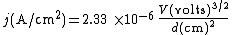Child-Langmuir law

Also found in: Dictionary.

Child-Langmuir law

A law governing space-charge-limited flow of electron current between two plane parallel electrodes in vacuum when the emission velocities of the electrons can be neglected. It is often called the three-halves power law, and is expressed by the equation below.Here V is the potential difference between the two electrodes, d their separation, and j the current density at the collector electrode, or anode. The potential difference V is the applied voltage reduced by the difference in work function of the collector and emitter. The Child-Langmuir law applies, to a close approximation, to other electrode geometries as well. Thus for coaxial cylinders with the inner cylinder the cathode, it leads to a deviation from the true value of the current density of 13% at most. See Space charge

Site: Follow: Share:
Open / Close